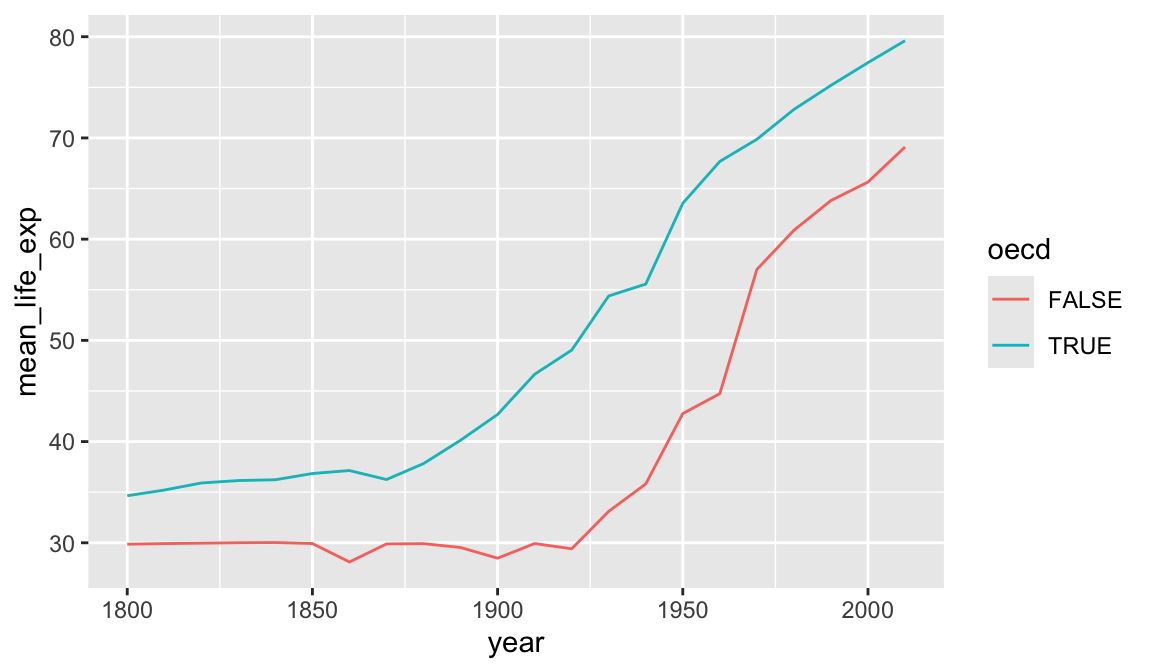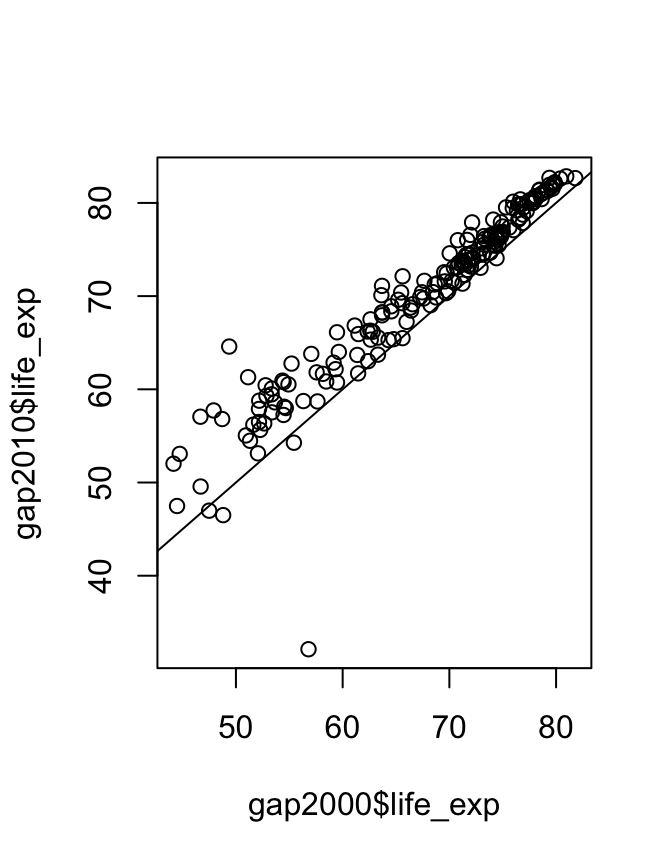# 4 Summarizing data

Having loaded and thoroughly explored a data set, we are ready to distill it down to concise conclusions. At its simplest, this involves calculating summary statistics like counts, means, and standard deviations. Beyond this is the fitting of models, and hypothesis testing and confidence interval calculation. R has a huge number of packages devoted to these tasks and this is a large part of its appeal, but is beyond the scope of today.

``````library(tidyverse)

gap_geo <- left_join(gap, geo, by="name")``````

## 4.1 Summary functions

R has a variety of functions for summarizing a vector, including: `sum`, `mean`, `min`, `max`, `median`, `sd`.

``mean( c(1,2,3,4) )``
``##  2.5``

We can use these on the Gapminder data.

``````gap2010 <- filter(gap_geo, year == 2010)
sum(gap2010\$population)``````
``##  6949495061``
``mean(gap2010\$life_exp)``
``##  NA``

## 4.2 Missing values

Why did `mean` fail? The reason is that `life_exp` contains missing values (`NA`).

``gap2010\$life_exp``
``````##    56.20 76.31 76.55 82.66 60.08 76.85 75.82 73.34 81.98 80.50 69.13 73.79
##   76.03 70.39 76.68 70.43 79.98 71.38 61.82 72.13 71.64 76.75 57.06 74.19
##   77.08 73.86 57.89 57.73 66.12 57.25 81.29 72.45 47.48 56.49 79.12 74.59
##   76.44 65.93 57.53 60.43 80.40 56.34 76.33 78.39 79.88 77.47 79.49 63.69
##   73.04 74.60 76.72 70.52 74.11 60.93 61.66 76.00 61.30 65.28 80.00 81.42
##   62.86 65.55 72.82 80.09 62.16 80.41 71.34 71.25 57.99 55.65 65.49 32.11
##   71.58 82.61 74.52 82.03 66.20 69.90 74.45 67.24 80.38 81.42 81.69 74.66
##   82.85 75.78 68.37 62.76 60.73 70.10 80.13 78.20 68.45 63.80 73.06 79.85
##   46.50 60.77 76.10    NA 73.17 81.35 74.01 60.84 53.07 74.46 77.91 59.46
##  80.28 63.72 68.23 73.42 75.47 65.38 69.74    NA 66.18 76.36 73.55 54.48
##  66.84 58.60    NA 68.26 80.73 80.90 77.36 58.78 60.53 81.04 76.09 65.33
##     NA 77.85 58.70 74.07 77.92 69.03 76.30 79.84 79.52 73.66 69.24 64.59
##     NA 75.48 71.64 71.46    NA 68.91 75.13 64.01 74.65 73.38 55.05 82.69
##  75.52 79.45 61.71 53.13 54.27 81.94 74.42 66.29 70.32 46.98 81.52 82.21
##  76.15 79.19 69.61 59.30 76.57 71.10 58.74 69.86 72.56 76.89 78.21 67.94
##     NA 56.81 70.41 76.51 80.34 78.74 76.36 68.77 63.02 75.41 72.27 73.07
##  67.51 52.02 49.57 58.13``````

R will not ignore these unless we explicitly tell it to with `na.rm=TRUE`.

``mean(gap2010\$life_exp, na.rm=TRUE)``
``##  70.34005``

Ideally we should also use `weighted.mean` here, to take population into account.

``weighted.mean(gap2010\$life_exp, gap2010\$population, na.rm=TRUE)``
``##  70.96192``

`NA` is a special value. If we try to calculate with `NA`, the result is `NA`

``NA + 1``
``##  NA``

`is.na` can be used to detect `NA` values, or `na.omit` can be used to directly remove rows of a data frame containing them.

``is.na( c(1,2,NA,3) )``
``##  FALSE FALSE  TRUE FALSE``
``````cleaned <- filter(gap2010, !is.na(life_exp))
weighted.mean(cleaned\$life_exp, cleaned\$population)``````
``##  70.96192``

## 4.3 Grouped summaries

The `summarize` function in `dplyr` allows summary functions to be applied to data frames.

``summarize(gap2010, mean_life_exp=weighted.mean(life_exp, population, na.rm=TRUE))``
``````## # A tibble: 1 × 1
##   mean_life_exp
##           <dbl>
## 1          71.0``````

So far unremarkable, but `summarize` comes into its own when the `group_by` “adjective” is used.

``````summarize(
group_by(gap_geo, year),
mean_life_exp=weighted.mean(life_exp, population, na.rm=TRUE))``````
``````## # A tibble: 22 × 2
##     year mean_life_exp
##    <dbl>         <dbl>
##  1  1800          30.9
##  2  1810          31.1
##  3  1820          31.2
##  4  1830          31.4
##  5  1840          31.4
##  6  1850          31.6
##  7  1860          30.3
##  8  1870          31.5
##  9  1880          32.0
## 10  1890          32.5
## # … with 12 more rows``````

### Challenge: summarizing

What is the total population for each year? Plot the result.

Advanced: What is the total GDP for each year? For this you will first need to calculate GDP per capita times the population of each country.

`group_by` can be used to group by multiple columns, much like `count`. We can use this to see how the rest of the world is catching up to OECD nations in terms of life expectancy.

``````result <- summarize(
group_by(gap_geo,year,oecd),
mean_life_exp=weighted.mean(life_exp, population, na.rm=TRUE))``````
``````## `summarise()` has grouped output by 'year'. You can override using the `.groups`
## argument.``````
``result``
``````## # A tibble: 44 × 3
## # Groups:   year 
##     year oecd  mean_life_exp
##    <dbl> <lgl>         <dbl>
##  1  1800 FALSE          29.9
##  2  1800 TRUE           34.7
##  3  1810 FALSE          29.9
##  4  1810 TRUE           35.2
##  5  1820 FALSE          30.0
##  6  1820 TRUE           35.9
##  7  1830 FALSE          30.0
##  8  1830 TRUE           36.2
##  9  1840 FALSE          30.0
## 10  1840 TRUE           36.2
## # … with 34 more rows``````
``ggplot(result, aes(x=year,y=mean_life_exp,color=oecd)) + geom_line()``A similar plot could be produced using `geom_smooth`. Differences here are that we have full control over the summarization process so we were able to use the exact summarization method we want (`weighted.mean` for each year), and we have access to the resulting numeric data as well as the plot. We have reduced a large data set down to a smaller one that distills out one of the stories present in this data. However the earlier visualization and exploration activity using `ggplot2` was essential. It gave us an idea of what sort of variability was present in the data, and any unexpected issues the data might have.

## 4.4 t-test

We will finish this section by demonstrating a t-test. The main point of this section is to give a flavour of how statistical tests work in R, rather than the details of what a t-test does.

Has life expectancy increased from 2000 to 2010?

``````gap2000 <- filter(gap_geo, year == 2000)
gap2010 <- filter(gap_geo, year == 2010)

t.test(gap2010\$life_exp, gap2000\$life_exp)``````
``````##
##  Welch Two Sample t-test
##
## data:  gap2010\$life_exp and gap2000\$life_exp
## t = 3.0341, df = 374.98, p-value = 0.002581
## alternative hypothesis: true difference in means is not equal to 0
## 95 percent confidence interval:
##  1.023455 4.792947
## sample estimates:
## mean of x mean of y
##  70.34005  67.43185``````

Statistical routines often have many ways to tweak the details of their operation. These are specified by further arguments to the function call, to override the default behaviour. By default, `t.test` performs an unpaired t-test, but these are repeated observations of the same countries. We can specify `paired=TRUE` to `t.test` to perform a paired sample t-test and gain some statistical power. Check this by looking at the help page with `?t.test`.

It’s important to first check that both data frames are in the same order.

``all(gap2000\$name == gap2010\$name)``
``##  TRUE``
``t.test(gap2010\$life_exp, gap2000\$life_exp, paired=TRUE)``
``````##
##  Paired t-test
##
## data:  gap2010\$life_exp and gap2000\$life_exp
## t = 13.371, df = 188, p-value < 2.2e-16
## alternative hypothesis: true mean difference is not equal to 0
## 95 percent confidence interval:
##  2.479153 3.337249
## sample estimates:
## mean difference
##        2.908201``````

When performing a statistical test, it’s good practice to visualize the data to make sure there is nothing funny going on.

``````plot(gap2000\$life_exp, gap2010\$life_exp)
abline(0,1)``````This is a visual confirmation of the t-test result. If there were no difference between the years then points would lie approximately evenly above and below the diagonal line, which is clearly not the case. However the outlier may warrant investigation.